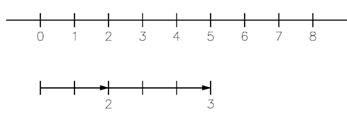## Adding Whole Numbers Assignment Help

Assignment Help: >> Basic Arithmetic Operations - Adding Whole Numbers

While numbers are added, the result is known as the sum.  The numbers added are known as addends. Addition is implies via the plus sign (+).  To addition explain the concept of addition; we will use a number line to graphically represent the addition of two numbers.

Example:        Add the whole numbers 2 and 3.

Solution:         By using a line which is divided into equal segments we can graphically show this addition.Beginning at zero, we first move two places to the right on the number line to represent the number 2.  We then move a further 3 places to the right to represent the addition of the number 3. The result corresponds to the position 5 on the number line.  Using this extremely basic approach we could see that 2 + 3 = 5.  Two rules govern the addition of whole numbers.